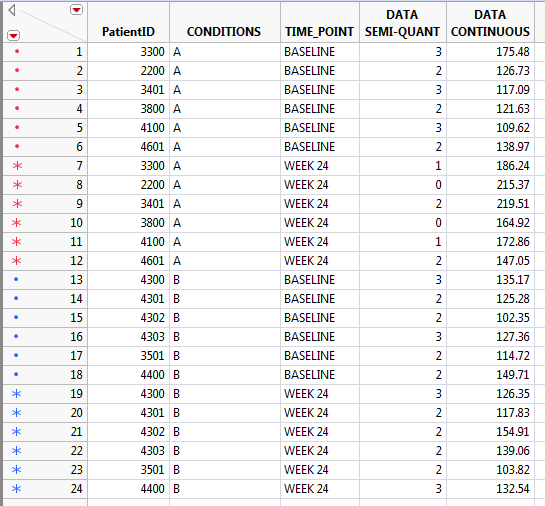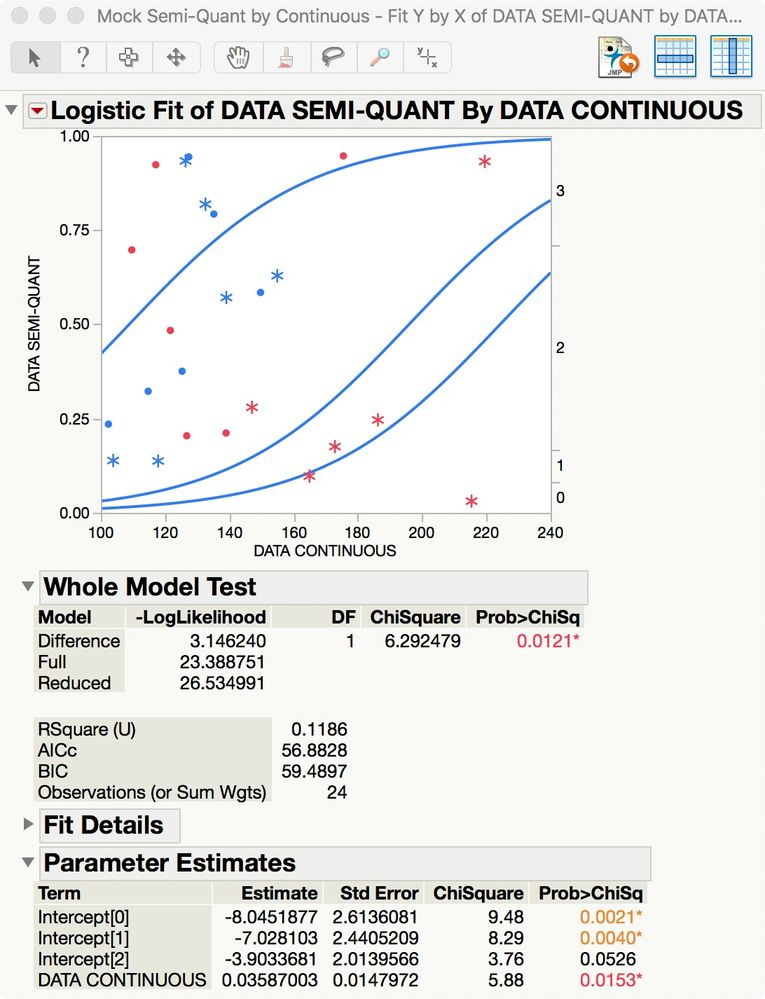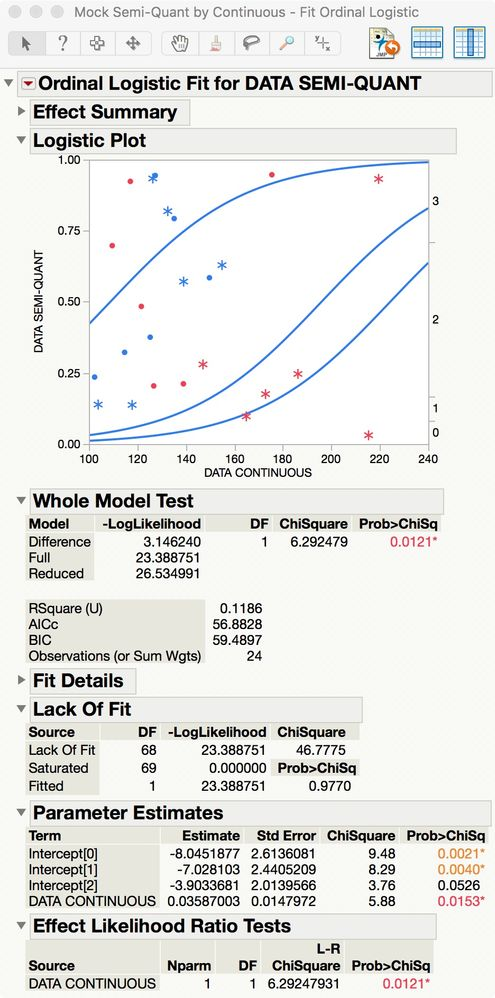Highlighted

## Semi-quant vs. continuous data: best method to explore relationship?

Hello again,

I need your advice about a rather basic question of statistics: what is the best method to explore the relationship (e.g. Spearman's correlation, Kendall's Tau, other) between semi-quantitative data (graded 0,1,2,3) and continuous variables (see table attached and screenshot)?Thanks

1 ACCEPTED SOLUTION

Accepted Solutions
Highlighted

## Re: Semi-quant vs. continuous data: best method to explore relationship?

I would start with ordinal logistic regression. Assuming that you have the ordinal modeling type for the DATA SEMI-QUANT response and the continuous modeling type for the DATA CONTINUOUS predictor/factor, you can use either Analyze > Fit Y by X or Analyze > Fit Model. The Logistic platform (from Fit Y by X launch) provides initial results like this:You can also get the ROC Curve and Lift Curve with this platform. Alternatively, you get these initial results from Ordinal Logistic Fit (from Fit Model launch):This platform allows more effects in the model such as other predictors and covariates and their transforms (interactions, powers). The Prediction Profiler and the same diagnostic plots are also available.

These regression techniques provide more information than simple measures of association or agreement.

Learn it once, use it forever!
Highlighted

## Re: Semi-quant vs. continuous data: best method to explore relationship?

I would start with ordinal logistic regression. Assuming that you have the ordinal modeling type for the DATA SEMI-QUANT response and the continuous modeling type for the DATA CONTINUOUS predictor/factor, you can use either Analyze > Fit Y by X or Analyze > Fit Model. The Logistic platform (from Fit Y by X launch) provides initial results like this:You can also get the ROC Curve and Lift Curve with this platform. Alternatively, you get these initial results from Ordinal Logistic Fit (from Fit Model launch):This platform allows more effects in the model such as other predictors and covariates and their transforms (interactions, powers). The Prediction Profiler and the same diagnostic plots are also available.

These regression techniques provide more information than simple measures of association or agreement.

Learn it once, use it forever!
Article Labels

There are no labels assigned to this post.